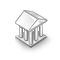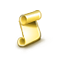• Get access

Interesting script?
So post a link to it -
let others appraise it

You liked the script? Try it in the MetaTrader 5 terminal# Statistics.mqh Functions - library for MetaTrader 5

Views:
8588
Rating:
Published:
2012.04.05 12:31
Updated:
2016.11.22 07:32

This library contains a set of basic statistical functions necessary for user data processing.

This library was first published in CodeBase at MQL4 - Statistica.mqh functions library. Some typos have been detected and corrected while transferring the functions to MQL5. The code has become more intuitively clear. Most functions have been written using the algorithms from S. Bulashov's book "Statistics for traders".

The library functions are as follows:

FunctionDescription
Mediana Median calculation
Mediana50 Median calculation by 50% interquantile range
Average Sample arithmetic mean calculation
Average50 Sample arithmetic mean calculation by 50% interquantile range
SweepCenter Sweep center calculation
AverageOfEvaluations Calculation of the average value of five upper evaluations
Variance Sample variance calculation
ThirdCentralMoment Third central moment calculation
FourthCentralMoment Fourth central moment calculation
Asymmetry Sample asymmetry calculation
Excess Sample excess calculation
Excess2 Another method of the sample excess calculation
Gamma Euler's gamma function calculation, x>0.
GammaStirling Euler's gamma function value calculation, for x>33 (Stirling's approximation)
VarianceOfSampleVariance Calculating the variance of a sample variance
VarianceOfStandartDeviation Calculating the variance of a standard deviation
VarianceOfAsymmetry Sample asymmetry variance calculation
VarianceOfExcess Sample excess variance calculation
VarianceOfAverage Sample mean variance calculation
Log Logarithm calculation
CensorCoeff Censoring ratio calculation
HistogramLength Calculating the optimal number of the histogram columns
Resize Calculating the optimal number of the array elements for the histogram
Histogram Creating the histogram to *.csv file
Cov Sample covariation calculation
Corr Sample correlation calculation
VarianceOfCorr Sample correlation variance calculation
AutoCorr Autocorrelation calculation
AutoCorrFunc Autocorrelation function calculation
aCoeff Calculating the a ratio in the linear regression equation (y=a*x+b)
bCoeff Calculating the b ratio in the linear regression equation (y=a*x+b)
LineRegresErrors Calculating the linear regression errors
eVariance Calculating the linear regression errors variance
aVariance Calculating the linear regression a parameter variance
bVariance Calculating the linear regression b parameter variance
DeterminationCoeff Determination ratio calculation
ArraySeparate Splitting the arr[n] array in two arrays
ArrayUnion Joining the two arrays into the array of arr[n] type
WriteArray Writing the one-dimensional array to *.csv file
WriteArray2 Writing the two-dimensional array to *.csv file

The file can be included in the projects requiring random sample parameters processing, its parameters evaluation, histograms etc.

Let's examine the call of some functions:

```//+------------------------------------------------------------------+
//|                                                         test.mq5 |
//|                        Copyright 2012, MetaQuotes Software Corp. |
//|                                              http://www.mql5.com |
//+------------------------------------------------------------------+
#property version   "1.00"

#include <Statistics.mqh>
//+------------------------------------------------------------------+
//| Script program start function                                    |
//+------------------------------------------------------------------+
void OnStart()
{
//--- specifying two values samples.
double arrX={3,4,5,2,3,4,5,6,4,7};
double arrY={7,4,1,2,1,6,9,2,1,5};
//--- calculating the mean
double mx=Average(arrX);
double my=Average(arrY);
//--- using the mean to calculate the variance
double dx = Variance(arrX,mx);
double dy = Variance(arrY,my);
//--- asymmetry value and excess
double as=Asymmetry(arrX,mx,dx);
double exc=Excess(arrX,mx,dx);
//--- covariation and correlation values
double cov=Cov(arrX,arrY,mx,my);
double corr=Corr(cov,dx,dy);
//--- showing results in the log file
PrintFormat("mx=%.6e",mx);
PrintFormat("my=%.6e",my);
PrintFormat("dx=%.6e",dx);
PrintFormat("dy=%.6e",dy);
PrintFormat("As=%.6e",as);
PrintFormat("exc=%.6e",exc);
PrintFormat("cov=%.6e",cov);
PrintFormat("corr=%.6e",corr);
}```

As you can see, most functions require the values (as input parameters) that can be calculated using other functions.

For example:

`double dx = Variance(arrX,mx);`

To calculate the variance, we have to calculate the mean at first. That gives a certain advantage regarding the calculations optimization. In case it is necessary to calculate the variance for several times, it will be better to find the mean once instead of doing it several times inside the function. That will save time.

This feature applies to most functions of the library.

Translated from Russian by MetaQuotes Software Corp.
Original code: https://www.mql5.com/ru/code/866Objects Description Activator

Enables the "Chart Objects Description" for non-programmers. For any open chart window.Price on Bollinger Channel

The indicator draws (in a separate window) the Bollinger Bands relative to the moving average and projection of the price bars.Percentage of Trend

This Indicator is used for calculating trend.ColorChart

Simple script to randomize all colors of your chart. Just click on it and see results yourself!